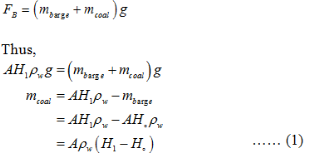# A Barge floating in fresh water....?

A Barge floating in fresh water…

(b)
The equation of the buoyant force on the empty barge is,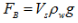The volume of the submerged surface in the water is,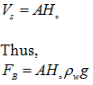( c)
The buoyant force is equal to force due to the weight of the barge.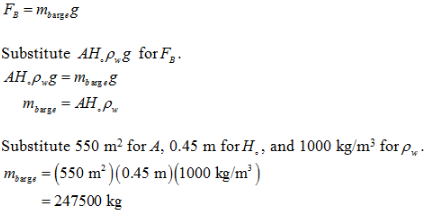(d)
There is no net force acting on the barge.

In first diagram, the net force acting is wrong. Thus, this diagram is wrong.

In second diagram, the net force acting is wrong. Thus, this diagram is wrong.

In third diagram. the magnitude ofthe buoyant force is not equal to the force due to the weight of the barge. Thus, this diagram is wrong.

In fifth diagram, there is no buoyant force acting on the barge.

In sixth diagram, the magnitude of the buoyant force is not equal to the force due to the diagram is weight of the barge.Thus, this diagram is wrong.

In fourth diagram shows the correct free body diagram. The buoyant force is equal and opposite to the force due to the weight of the barge.

Thus, fourth diagram is the correct diagram.

(e)
The expression for new buoyant force when coal is loaded in the barge is,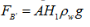The buoyant force is equal to force due to the of the barge and coal.